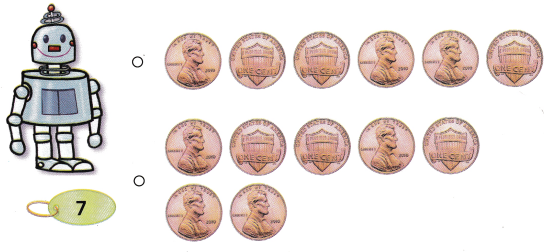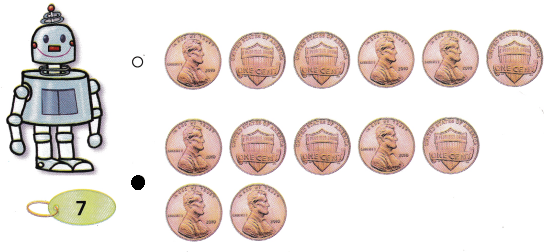# Texas Go Math Kindergarten Lesson 15.1 Answer Key Penny

Refer to our Texas Go Math Kindergarten Answer Key Pdf to score good marks in the exams. Test yourself by practicing the problems from Texas Go Math Kindergarten Lesson 15.1 Answer Key Penny.

## Texas Go Math Kindergarten Lesson 15.1 Answer Key Penny

Essential Question
How do you identify, describe, and name pennies?

Explore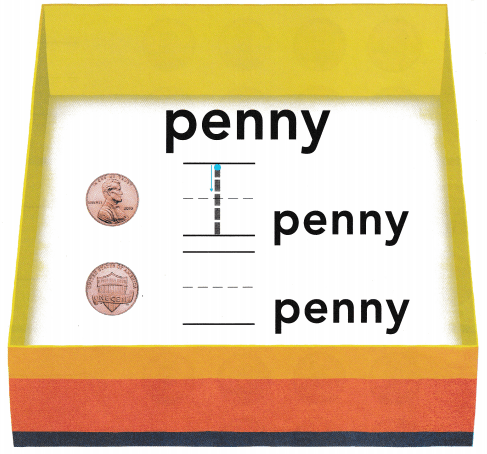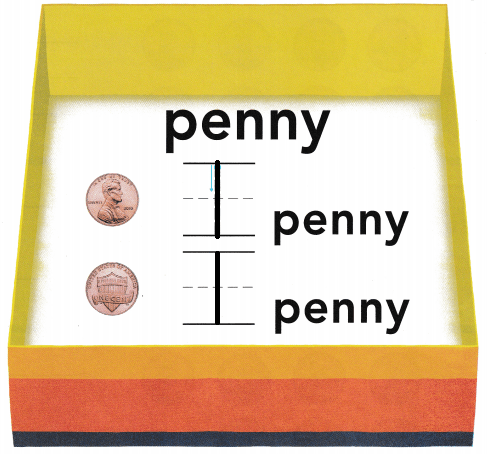Explanation:
1 penny = 1 cent

Directions
Place a penny to march each one shown. Tell what is alike about the pennies. Tell what is different about the pennies. Trace or write the number that shows how many pennies.

Share and Show

Question 1.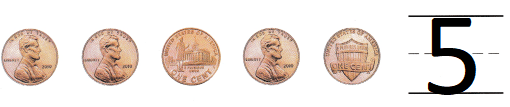Explanation:
a small sum of money.
There are 5 pennies

Question 2.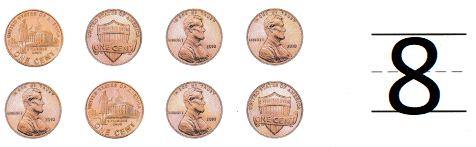Explanation:
a small sum of money.
There are 8 pennies

Question 3.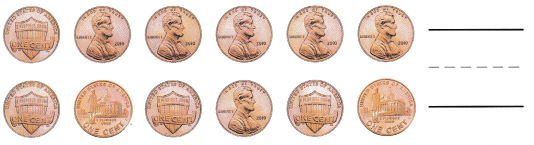Explanation:
a small sum of money.
There are 12 pennies

Question 4.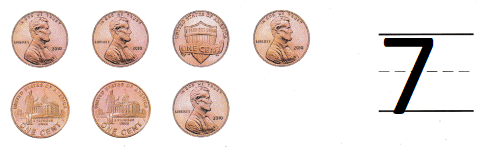Explanation:
a small sum of money.
There are 7 pennies

Directions
1-4. Count the pennies. Write the number that shows how many pennies.

Question 5.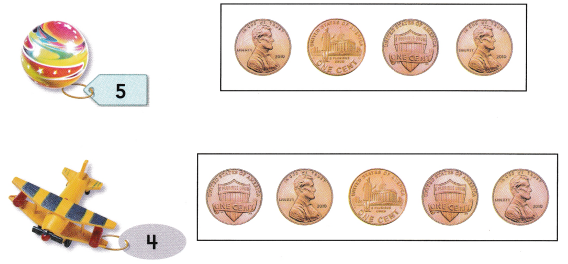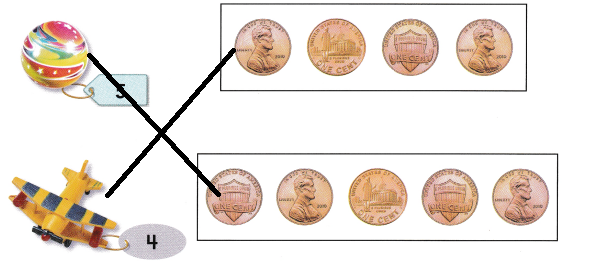Explanation:
A marble cost 5 pennies
and a helicopter costs 4 pennies

Question 6.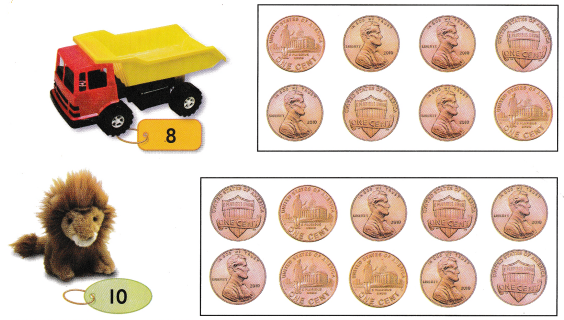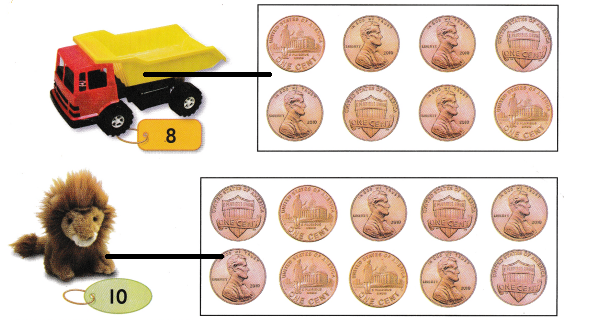Explanation:
A truck costs 8 pennies
and a lion toy costs 10 pennies.

Directions
5-6. Draw a line to match the toy to the pennies needed to buy the item.

Home Activity

• Show your child a set of 1 to 5 pennies. Have your child tell you the name of the coin and count the number.

Problem Solving

Question 7.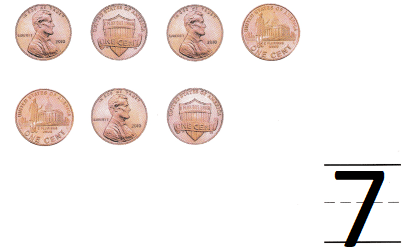Explanation:
a small sum of money.
There are 7 pennies

Question 8.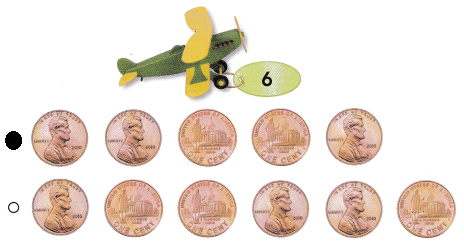Explanation:
a small sum of money.
The toy helicopter toy costs 6 pennies

Directions
7. Chloe buys a new toy with four pennies. Circle the number of pennies she needs. Write the number. 8. Choose the correct answer. Which set of pennies shows the number you need to buy the plane?

### Texas Go Math Kindergarten Lesson 15.1 Homework and Practice

Question 1.Explanation:
a small sum of money.
There are 9 pennies

Question 2.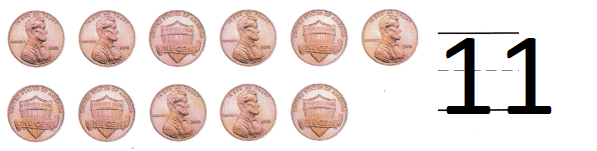Explanation:
a small sum of money.
There are 11 pennies

Question 3.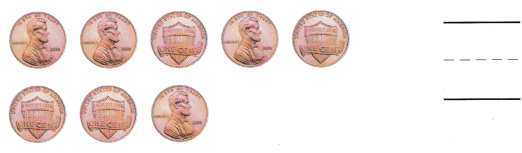Explanation:
a small sum of money.
There are 8 pennies

Directions
1-3. Count the pennies. Write the number that shows how many

Texas test Prep

Question 4.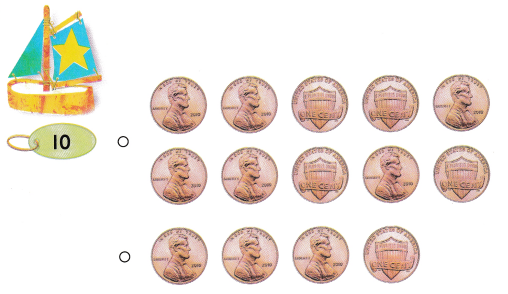Explanation:
a small sum of money.
The toy costs 10 pennies

Question 5.